# Monotonic Functions, Inequalities, and Optimization

Looking for a cluster of questions on similar topics, I found several from this year in which monotonic functions (functions that either always increase, or always decrease) provide shortcuts for various types of problems (optimization with or without calculus, and also algebraic inequalities). We’ll look at a few of these.

## Finding a minimum without calculus – but with three monotonic functions

This question came from Amia in March:

Hi Dr math,

I have a question about minimizing the function below.

Can you help me to understand it?

Minimize the function √(ex^2 – 1).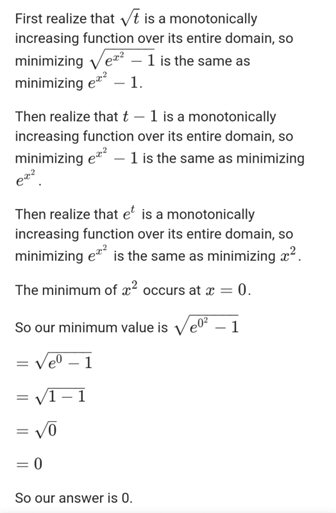Doctor Fenton answered, filling in some gaps in the solution:

Hi Amia,

You said you have a question, but your attachment is only a solution of the problem, and it gives reasons for each step.  What is your question?  Which step of the argument are you asking for help on?

The only step I see which might be confusing is the third statement, that exp(x2) has a minimum at x = 0 because x2 has a minimum at x = 0.  The first two steps are based on the fact that if f is strictly increasing and g is strictly increasing, then the composition f(g(x)) is also strictly increasing.

In the third statement, exp(x2) is not strictly increasing, nor is x2.  However, the range of g(x) = x2 is the non-negative real numbers, and g is strictly increasing on [0,∞).  [It’s also true that if g is strictly decreasing, then f(g(x)) will also be strictly decreasing.]

x2 is strictly decreasing on (-∞,0] and strictly increasing on [0,∞), so exp(x2) is also strictly decreasing on (-∞,0] and strictly increasing on [0,∞).

Let’s look at graphs for the three steps in the solution.

Our function is $$\sqrt{e^{x^2}-1}=f(g(h(k(x)))),$$ where $$f(x)=\sqrt{x}\\g(x)=x-1\\h(x)=e^x\\k(x)=x^2.$$

The first three functions are monotonically increasing on their domains; here are our $$f(x)=\sqrt{x}$$ (red), $$g(x)=x-1$$ (orange), and $$h(x)=e^x$$ (green):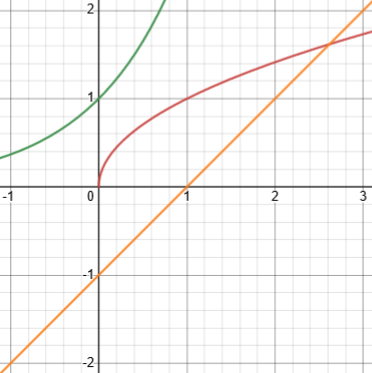Here is the graph of $$k(x)=x^2$$ (green), and of the entire composite function $$f(g(h(k(x))))=\sqrt{e^{x^2}-1}$$ (red):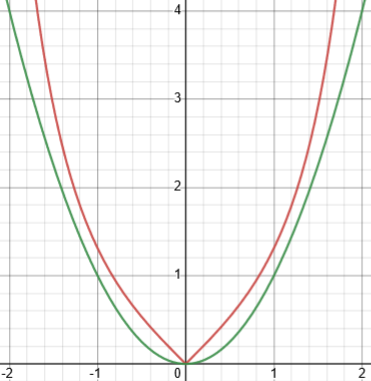Clearly, the minimum is at zero. The same would be true for $$\sqrt{e^{x^2}\mathbf{+}1}$$, where I changed the subtraction to addition to get the minimum away from the origin: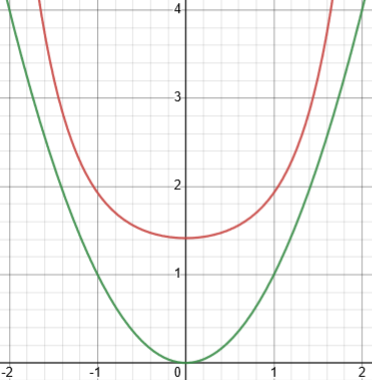Doctor Rick expanded on the general principle illustrated here:

Hi, Amia. I’d like to add that the key idea here is one I have used frequently to simplify finding, for example, the minimum distance of a point (x, y) from the origin. The distance is √(x2 + y2), but because the square-root function is monotonically increasing, it suffices to minimize (x2 + y2). It can be a great help not to need to deal with the square root!

If a function g is a monotonically increasing function, then a value of x that (locally/globally) (minimizes/maximizes) f(x) also (locally/globally) (minimizes/maximizes) g(f(x)), and vice versa.

How do I know this? Consider the case of local minima as an example. A monotonically increasing function is a function g for which

if x1 ≤ x2 then g(x1) ≤ g(x2).

Note that a monotonically increasing function is necessarily invertible, and its inverse is also monotonically increasing. Therefore the implication above goes both ways:

x1 ≤ x2 if and only if g(x1) ≤ g(x2).

If x0 is a local minimum of a function f, then there exists an interval around x0 on which f(x0) ≤ f(x). But this implies that g(f(x0)) ≤ g(f(x)) on that interval, and thus x0 is a local minimum of g(f(x)).

Your problem takes this idea and runs with it, applying it several times to make the minimization problem trivial! That won’t happen often.

Usually monotonic functions will just make things easier, rather than eliminate all the work, as it did here. The next example is more typical, similar to the example of minimizing the square of a distance.

## Finding a maximum with calculus – and a monotonic function

Amia wrote again in May:

Hi Dr math,

I have below solved optimization problem.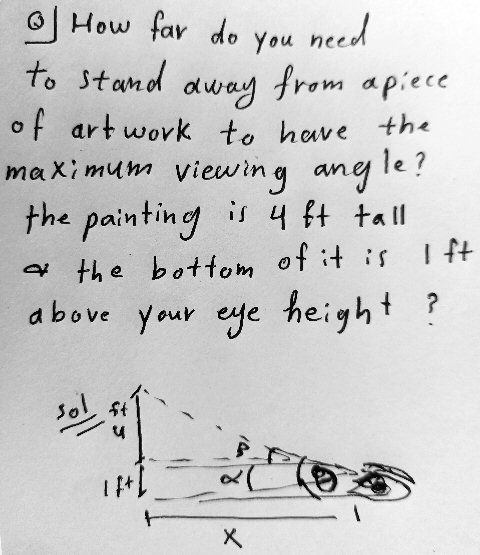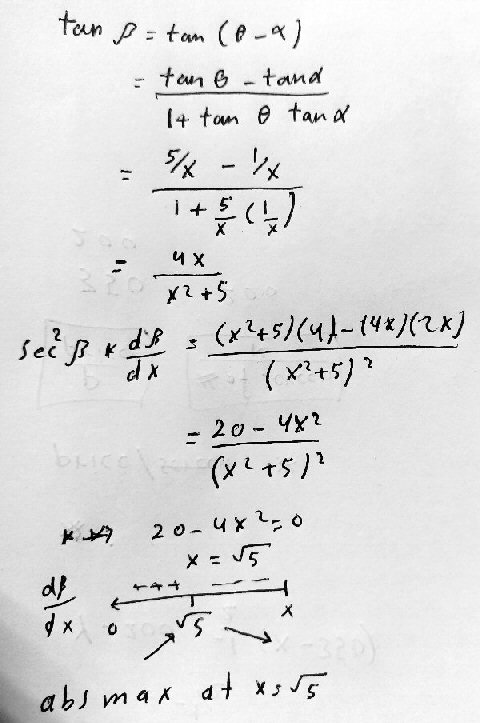In last steps, when I differentiate, I’ve got sec2β. dβ/dx = (20 – 4x2)/(x2 + 5)2,

Why we don’t divide both sides by sec2β, then make dβ/dx = zero?

Here is a clearer picture, showing the maximum angle β and all the angles mentioned, to scale: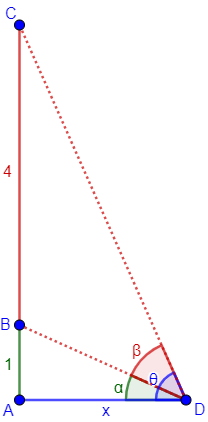Amia expressed the tangent of angle β, the difference of two angles, in terms of x, and differentiated both sides. (Alternatively, he could have expressed angle β itself as an inverse tangent, making a particularly complicated derivative.) Then, as I initially saw it, he mentally went from $$\sec^2(\beta)\frac{d\beta}{dx}=\frac{20-4x^2}{(x^2+5)^2}$$ to $$\frac{d\beta}{dx}=\frac{20-4x^2}{\sec^2(\beta)(x^2+5)^2}$$ and set the numerator to zero.

I answered, missing the point that there was no actual division in the work shown:

Hi, Amia.

“Why don’t we divide ..,?” That appears to be what you did! If you did something else, please explain what you actually did, and why?

I see nothing wrong with doing what you are asking about, since sec2(β) is never zero.

Without explicitly dividing, you can say that dβ/dx = 0 if and only if the LHS is zero, and the RHS is zero if and only if the numerator is zero, which you proceed to do.

The last comment reflects what was actually written. But it’s still reading into it something that Amia had not thought.

Amia wrote out the suggested alternative, which was more complicated than what I thought: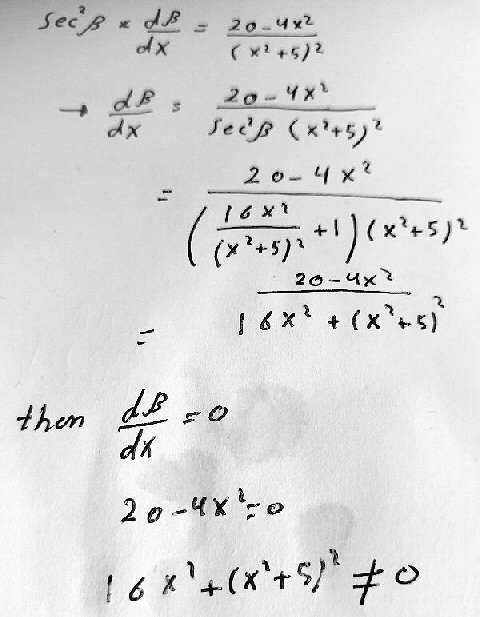Doctor Rick answered (I’d been busy all day and unable to respond):

Thanks, Amia, now we can see what you were thinking: Why don’t we go to all the trouble of expressing sec2 β in terms of x so that we can divide both sides by that expression in x?

When someone asks, “Why don’t we do it this other way?“, we will often respond, “Try it and see!” You have tried it! What do you see?

What I see is that, after doing all this work, you end up ignoring it anyway, because only the numerator matters in finding the zeros of a rational expression. (Well, it also matters that the denominator is not zero, but we could tell that sec2 β ≠ 0 without rewriting it – the minimum value of sec2 β is 1.)

I had found it so natural to just set the numerator equal to zero that I had thought of that as a division (that didn’t need to be written out). But it isn’t quite so obvious to a student – a good lesson to be reminded of.

Now Doctor Rick brings in the theme of the present post:

Personally, I would have done something simpler. We had the equation

tan β = 4x/(x2 + 5) .

We want to maximize β, right? At this point I would simply note that the tangent function is monotonically increasing, so that to maximize β, we just have to maximize tan β, that is, maximize f(x) = 4x/(x2 + 5). This is a very useful trick.

The largest value of the angle corresponds to the largest tangent, so we can ignore the monotonic tangent function. (We could also express this in terms of a composite with a monotonic function, namely $$\beta=\arctan\left(\frac{4x}{x^2+5}\right)$$, and think  just as in the previous problem.)

## Finding a maximum with the AM-GM inequality – and a couple monotonic functions

Two days later, Amia had this question about yet another way to optimize, which will involve just a little application of the monotonic principle:

Hi Dr math,

I use the AM-GM inequality to solve the question below,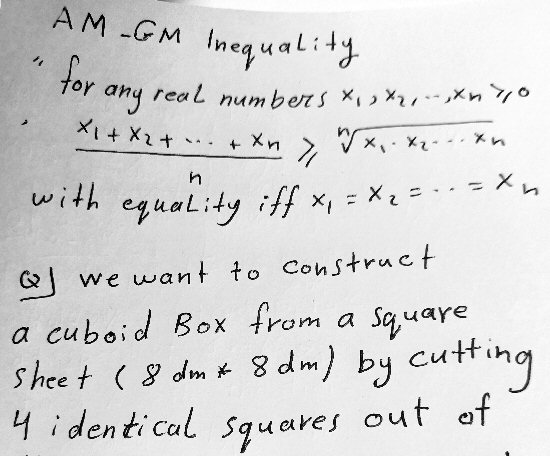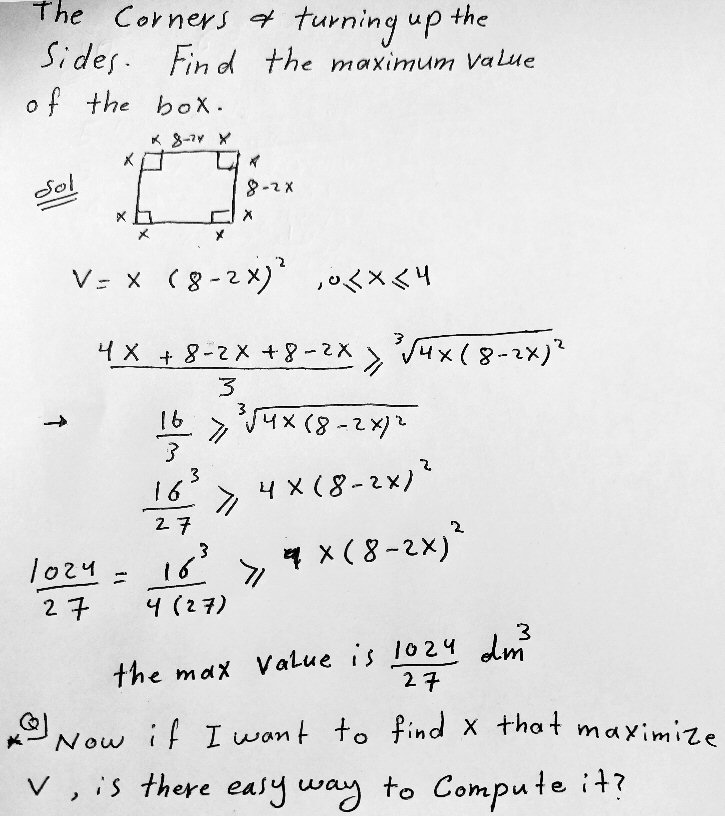But when I want to compute x, I see it is complicated, you need to solve 1024/27 = x(8-2x2).

Is there any easy way?

The AM-GM inequality says that the Arithmetic Mean (sum divided by n) of any set of n numbers is at least as great as the Geometric Mean (nth root of the product), being equal only when the numbers are all the same. This is something I was not exposed to in my education as much as students in other countries seem to be.

Amia has used that to relate the cube root of the volume, which is a product of three factors, to the average of those three factors. This provides an upper bound on the volume. There is one step that is unexplained here, which we’ll get back to: Where did the 4 come from in the first factor?

Doctor Rick gave a quick hint:

Hi, Amia.

Look again at the AM-GM Theorem. Under what conditions does equality hold?

Amia replied,

For positive real numbers.

… and then, 6 minutes later,

When 4x = 8 – 2x,

6x = 8

x = 4/3

This is the solution. The volume will equal this upper bound when the three numbers being averaged, $$4x$$, $$8-2x$$, and $$8-2x$$ are equal. Solving for x allows us to verify that the maximum can actually be attained.

Doctor Rick responded:

Good, on further thought you realized what I was getting at. Since you have previously gotten the answer by other means, you know your answer is correct.

Amia had discussed with us how to solve the same problem by differentiation a couple days before.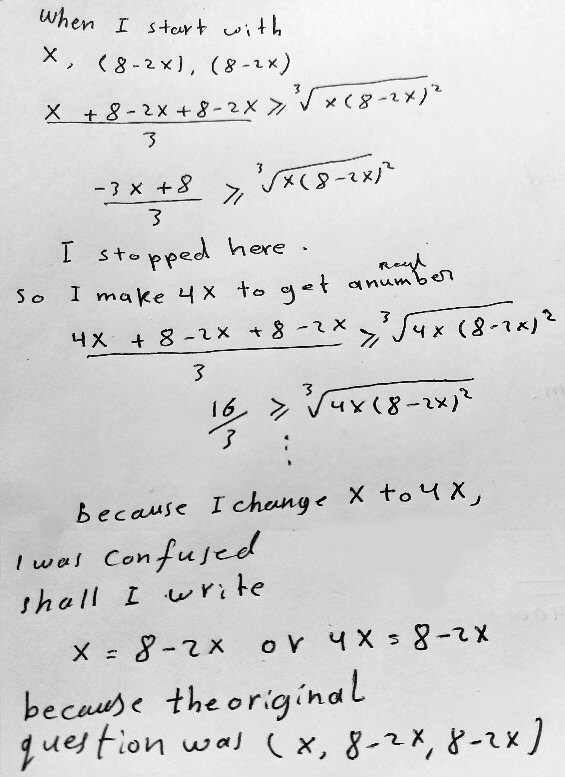Doctor Rick responded:

There’s a word you wrote above “a number” that I can’t make out. Does that word explain why you replaced x with 4x?

As I see it, you saw that (since f(x) = x3 is a monotonically increasing function) you can maximize V by maximizing V1/3 = 3√(x(8–2x)2). Viewing this expression as a geometric mean, you applied the AM-GM Theorem to it, obtaining

3√(x(8–2x)2) ≤ (x + 2(8–2x))/3 = (16–3x)/3                

But that wasn’t helpful, so you looked for a way to get the RHS to be a constant. Somehow you hit on this:

(4V)1/3 = 3√(4x(8–2x)2) ≤ (4x + 2(8–2x))/3 = 16/3    

However you came up with that, it’s good.

I tend to think of these “Use AM-GM to …” problems as tricks, which perhaps someone once discovered, perhaps by accident, that are now passed along in certain mathematical cultures. Here, we can multiply the volume by 4 (another monotonic function!) and optimize that, which works well because now the sum of the three factors is constant, yielding a fixed upper bound.

Then, on the question at the bottom (“because I change …”):

What matters here is the inequality , which we know to be true by the AM-GM Inequality. According to that theorem, the equality holds (that is, the LHS is maximized) when

4x = 8 – 2x

from which you obtained the solution x = 4/3. That is correct.

Perhaps you yourself do not know why you introduced the 4. (Is this someone else’s work, or modeled after someone else’s work on a similar problem, rather than all your own idea?) If you look above, I was careful to note exactly how the introduction of the 4 is justified: the new cube root expression is the cube root of 4 times the volume of the box we’re making, and maximizing this will maximize V itself. We are not “changing x to 4x” as you put it; x still represents the height of the box.

This illustrates the importance of understanding why you do each step in a solution; just copying ideas or following a routine can leave you unsure of the meaning of your result.

## Rules for solving inequalities using monotonic functions

We’ll close with a non-Amia question from June:

I am investigating properties of equality (and inequality)

There are many discussions of the addition, subtraction, multiplication, and division properties of equality.

Can you point me to a discussion that includes properties with respect to (e.g.) inversion, exponentiation, the taking of logarithms?

I would expect to see “you can invert both sides of an equality and still have an equality; inverting both sides of an inequality reverses the sense of the inequality” among the matters discussed. “Any root” and “any power” should be there too. You get the idea.

I’d like such a discussion for myself and (within reason) for interested and able students.

Thanks for any direction you can provide.

I answered briefly, late at night:

Hi, Peter.

I can give a quick (partial) answer: This is covered [as far as equations are concerned] in our article

Principles for Solving an Equation

In particular, in the section “Applying a function to each side”, I say,

… we don’t need a new property if we want, say, to take the logarithm of both sides of an equation. We just need to know that the logarithm of any given number is always the same (so that the result, if two expressions are equal, is two logs that are equal), and to be aware that we have to check that our solution is not invalid (extraneous).

You could call this the “function property of equality”, but as I say, it’s really a property of functions rather than of equality: the function must be well-defined.

Then I go on to talk about the need for equivalence, which is the other side of this: For some purposes, it is necessary that the function be invertible. That’s why squaring, for instance, needs special treatment, because now you can get extraneous solutions.

Now, this doesn’t include inequalities. There, I think you’d also need to consider things like monotonicity. I’ll give that a little more thought (and maybe others of us will have specific ideas).

The next day, after sleeping on it, I filled in those details for inequalities:

I’ll add a little on inequalities, though it is just an expansion of what I said: Monotonicity is the key.

Multiplying by a positive number, adding any number, cubing, taking the log to any base greater than 1, and exponentiating with any base greater than 1 will all preserve the sense (direction) of an inequality, because the functions being applied (ax, x+a, x3, loga(x), ax) are all monotonically increasing functions. That is, if x < y, then f(x) < f(y). For example, 4 < 8, and log2(4) < log2(8).

Multiplying by a negative number, taking the log to a base between 0 and 1, and exponentiating with any base between 0 and 1 will all reverse the sense of an inequality, because these functions (ax, loga(x), ax) are all monotonically decreasing functions. That is, if x < y, then f(x) > f(y). For example, 4 < 8, and log1/2(4) > log1/2(8).

But a function that is not monotonic, such as f(x) = x2can’t be applied to an inequality, unless you have an additional condition (such as that both sides are known to be positive). For example, -1 < 2, and (-1)2 < 22; but -2 < 1, and (-2)2 > 12.

Note that f(x) = 1/x is decreasing for positive x and for negative x, but not for all x, so when you take the reciprocal of an inequality will reverse the sense as long as both sides are known to have the same sign: 1 < 2 and 1/1 > 1/2 (reversed), whereas -1 < 2, and 1/(-1) < 1/2.

So the rule is the same as for equations, with the addition of monotonicity.

So, for example, you can’t square both sides of an inequality unless you know both sides have the same sign. And the same is true for taking the reciprocal.

This site uses Akismet to reduce spam. Learn how your comment data is processed.